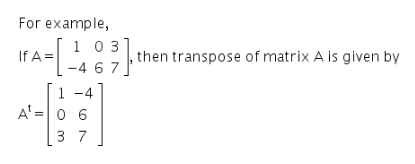# Define transpose of a matrix with an example

Define transpose of a matrix with an example.

Transpose of a matrix is the matrix obtained on interchanging its rows and columns.
If A is a matrix, then its transpose is denoted by At.